# Bathymetry from wave propagation analysis

Article reviewed by

It is possible to determine the bathymetry of a shallow coastal areas using radar data. This article discusses measurements carried out during storm conditions off the Island of Sylt in the German Bight. Radar data are analyzed through inverse application of the non-linear and linear wave theory; observed wave characteristics are used to derive information on water depths and currents. Radar techniques for the detection of wave characteristics are explained in the article Use of X-band and HF radar in marine hydrography.

## Introduction

The determination of the bathymetry in coastal environments by utilizing the ocean wave-shoaling photographic imagery and the observed reduction of ocean wave phase speed with decreasing water depth, is used since the WW-II (Williams 1946). The development of various ground-based observation instruments (mainly radar and video imagery) and the exponential increase of computational power have promoted new methodologies for bathymetry survey based on observation of the sea surface, e.g. Bell 1999, Seemann et al. 1999, Stockdon and Holman 2000, Dankert 2003, Bell et al. 2004, Catalan and Haller 2008, Senet et al. 2008. The core of these methods is the inverse use of linear or non-linear models for the propagation of the wavefield over an uneven seabed, in order to derive information on water depths and currents.

As an illustration we discuss here twelve hourly radar datasets acquired during storm conditions, which are analyzed with two methods: (1) the non-linear method of Bell et al. 2004 (henceforth BW04), which is based on inversed application of the non-linear wave dispersion equation of Hedges (1976) and (2) the Dispersive Surface Classificator (henceforth DiSC08, Senet et al. 2008), which is based on inversion of the linear wave theory. The performance of the two methods is assessed by comparing the resulting bathymetries to an echo sounder survey.

## Area of investigation and data acquisition

The radar was mounted close to the lighthouse List West on the Island of Sylt in the German Bight. The acquired radar images cover the tidal inlet at List West, the Lister Landtief (maximum depth 11m), and parts of the Lister Tief (maximum depth 40m). The coastal waters around List West were observed because of the extremely high morphodynamic activity. A sand bar between the Lister Landtief and the Lister Tief is in the process of breaking, which leads to a profound change of the local tidal-stream situation; the tidal range of the area is 2m.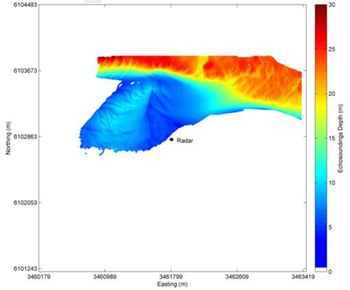Figure 1. Bathymetry of area of investigation acquired by multibeam echosounder.

The instrument used for the acquisition of sea-surface image sequences is a ground-based nautical X-band radar with horizontal polarization. The device utilized during the experiments is a software–hardware combination consisting of a commercial, navigational Furuno X-band radar antenna and radar device, a WaMoS II analog–digital converter and acquisition of radar image sequences software. The radar was mounted from 1996 to 2007; the image sequences of the present investigation were recorded hourly from 23:00 UTC on 26.08.2003 to 10:00 UTC on 27.08.2003. During the data acquisition the minimum and maximum wind speed were 12 m/s and 17 m/s, respectively and the minimum and maximum significant wave height, 1.2 m and 1.6 m respectively, as measured by deployed instruments in the area of investigation. For the determination of datum, tidal gauge measurements were also used. A dataset of the bathymetry of part of the radar-covered area was acquired by multibeam echo sounder (by KOK/GKSS) on 25th of August 2003 (Fig. 1); the spatial resolution of the bathymetric data set is 2m x 2m and has been averaged over the grid of the radar results.

## Data analysis

The BW04 and DiSC methods have common steps of analysis which are presented shortly in the current paragraph. The analysis of both methods begins with the conversion from the polar coordinates in which the raw radar data are recorded to a georeferenced Cartesian grid. The location of the transmitter and hence the origins of the polar conversions were determined by DGPS. A Fourier transform was carried out on each pixel through time in the image sequences to isolate individual wave frequencies. Each frequency layer within the transform was then analyzed to determine the variations in wavelength across the area viewed by the radars, using a discrete 2-D Fourier transform technique that isolates the strongest wave signal in the sub-images. Small subsections of the Fourier layer were used for this analysis. The wavelengths calculated from this analysis were then used in a least squares fit to find the water depth at each pixel, calculated both from linear wave theory (DiSC) and from a non-linear wave dispersion equation (BW04). For an explanation of these theories, see the article Shallow-water wave theory.

## Results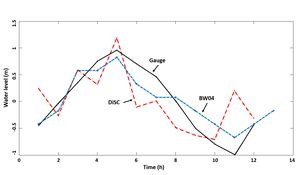Figure 2. Time series of water level calculated with BW04, DiSC in the deepest part of the tidal channel together with the measured water level.

The results of the analysis are 12 hourly bathymetries from both methods. The 12-hour average is shown in Fig. 3 for the BW04 method. The spatial resolution for BW04 is 60m and for the DiSC is 40m. The time series of instant water depth in the deepest point from which the mean of each time series was subtracted, are plotted together with the tide gauge data in Fig. 2. The comparison of the hourly water levels with the tidal gauge data demonstrates that although none of the two methods is precise for the determination of the water level, the results of BW04 have higher correlation with the measured water level, due to the used model. The Hedge’s dispersion function includes the significant wave height; therefore the “noise” of the actual wave conditions is reduced in comparison of the linear dispersion used in DiSC.

Comparison of the BW04 and DiSC bathymetries to the echo sounder data reveals the relative errors of the BW04 (Fig. 4) and DiSC (Fig. 5) methods.Figure 3. Averaged bathymetry over 12h obtained from the BW04 method.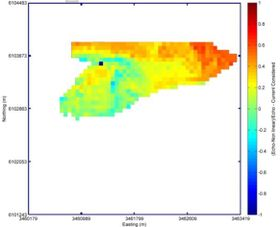Figure 4. Map of the relative error of the BW04 method.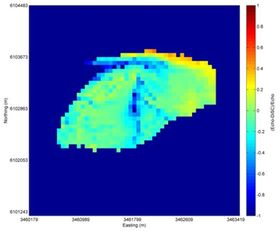Figure 5. Map of the relative error of the DiSC method.

## DiscussionFigure 6. Scatter plot of the BW04 depth compared to the surveyed depth.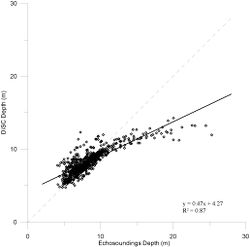Figure 7. Scatter plot of the DiSC depth compared to the surveyed depth.

The depths obtained from the two inversion methods were corrected with the measured tidal level. The results are compared to the echo sounder data in Figs. 6 and 7.

The scatter plots for both methods demonstrate clustering of the results. The first cluster lays between 4.5m (the lower limit depends on the available echosoundings bathymetry) and 10m for BW04 and 12m for DiSC. At this cluster both methods present significant correlation (1 by 1) with the surveyed bathymetry. The second cluster is formed from the depths greater than 12m, where the depth is clearly underestimated. The underestimation of the deeper areas is the main reason for the inclination of the trend lines from the y=x line.

The spatial distribution of the error illustrates the main sources of the error. In both cases, the bathymetry for deeper areas than 12m is underestimated. This is due to the lack of sufficiently long waves; the wave propagation celerity does not strongly depend on depth for wavelengths smaller than $2 \pi \times$ depth. The bathymetric gradient is the second source of the error. Both methods fail to determine the bathymetry over the steep sides of the ship channel and at the areas with sand dunes. This is obvious by comparing the map of the high resolution survey (Fig. 1) with the error maps. The BW04 approach has higher accuracy at the shoals due to the adapted wave model, but the mean accuracy of the DiSC is higher (approximately 90%) than the BW04 (more than 80%).

The above example shows that in principle, the inversion of the wave field propagation for the determination of the depth is a reasonable method with significant results for shallow coastal areas and it could be used in operational base at hot spots of crucial sediment motion, such as Sylt.